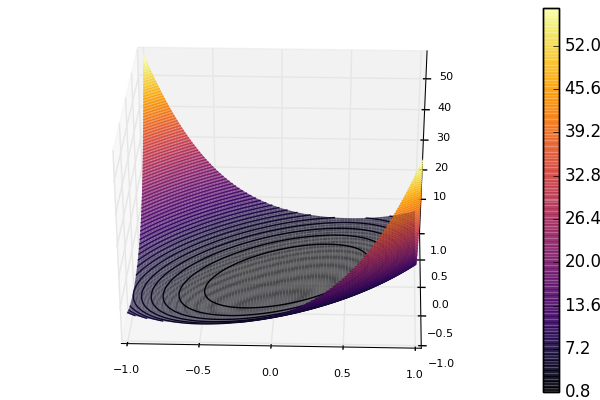# Preconditioning

The GradientDescent, ConjugateGradient and LBFGS methods support preconditioning. A preconditioner can be thought of as a change of coordinates under which the Hessian is better conditioned. With a good preconditioner substantially improved convergence is possible.

A preconditioner Pcan be of any type as long as the following two methods are implemented:

• A_ldiv_B!(pgr, P, gr) : apply P to a vector gr and store in pgr (intuitively, pgr = P \ gr)
• dot(x, P, y) : the inner product induced by P (intuitively, dot(x, P * y))

Precisely what these operations mean, depends on how P is stored. Commonly, we store a matrix P which approximates the Hessian in some vague sense. In this case,

• A_ldiv_B!(pgr, P, gr) = copy!(pgr, P \ A)
• dot(x, P, y) = dot(x, P * y)

Finally, it is possible to update the preconditioner as the state variable x changes. This is done through precondprep! which is passed to the optimizers as kw-argument, e.g.,

   method=ConjugateGradient(P = precond(100), precondprep! = precond(100))


though in this case it would always return the same matrix. (See fminbox.jl for a more natural example.)

Apart from preconditioning with matrices, Optim.jl provides a type InverseDiagonal, which represents a diagonal matrix by its inverse elements.

## Example

Below, we see an example where a function is minimized without and with a preconditioner applied.

using ForwardDiff
initial_x = zeros(100)
plap(U; n = length(U)) = (n-1)*sum((0.1 + diff(U).^2).^2 ) - sum(U) / (n-1)